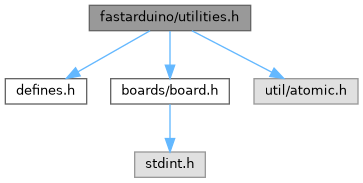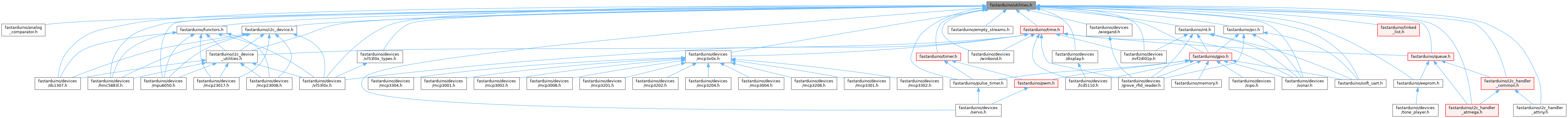FastArduino  v1.8 C++ library to build fast but small Arduino/AVR projects
utilities.h File Reference

General utilities API that have broad application in programs. More...

`#include "defines.h"`
`#include "boards/board.h"`
`#include <util/atomic.h>`
Include dependency graph for utilities.h:This graph shows which files directly or indirectly include this file:Go to the source code of this file.

## Namespaces

utils
Contains all generic utility methods.

## Macros

#define synchronized   _Pragma("GCC diagnostic ignored \"-Wreturn-type\"") ATOMIC_BLOCK(ATOMIC_RESTORESTATE)
Make a block of code a critical section (not interruptible). More...

## Enumerations

enum  utils::UnitPrefix : int8_t {
GIGA = 9,
MEGA = 6,
KILO = 3,
HECTO = 2,
DECA = 1,
NONE = 0,
DECI = -1,
CENTI = -2,
MILLI = -3,
MICRO = -6,
NANO = -9
}
Common prefixes for measurement units. More...

## Functions

template<typename T >
constexpr T utils::constrain (T value, T min, T max)
Constrain `value` to be greater than or equal to `min` and lower than or equal to `max`. More...

template<typename TI , typename TO >
constexpr TO utils::map (TI value, TI input_min, TI input_max, TO output_min, TO output_max)
Linearly transform `value` from range [`input_min` ; `input_max`] to range [`output_min` ; `output_max`]. More...

constexpr uint32_t utils::power_of_10 (int8_t n)
Calculate a power of 10 at compile-time, provided that `n` is a constant at call time. More...

constexpr int16_t utils::map_raw_to_physical (int16_t value, UnitPrefix prefix, int16_t range, uint8_t precision_bits)
Convert the raw `value`, obtained from an electronics device, using `precision_bit` number of bits (that defines the input range) into a physical measure for which `range` defines the complete output range for such value, adjusted according to the unit `prefix` that we want in the resulting measure. More...

constexpr int16_t utils::map_physical_to_raw (int16_t value, UnitPrefix prefix, int16_t range, uint8_t precision_bits)
Convert an absolute physical `value`, expressed in some given measurement unit, scaled with `prefix`, into a raw measurement as if obtained from a electronics device, using `precision_bit` number of bits (that defines the device raw measure range); for this device, physical measures are within `range`. More...

constexpr uint8_t utils::low_byte (uint16_t word)
Extract the low order byte of a 16-bits word.

constexpr uint8_t utils::high_byte (uint16_t word)
Extract the high order byte of a 16-bits word.

constexpr uint16_t utils::as_uint16_t (uint8_t high, uint8_t low)
Convert 2 bytes into an unsigned int. More...

template<typename T >
constexpr T utils::is_zero (T value, T default_value)
Replace `value` by `default_value` if not "true" (also known as "Elvis operator"). More...

template<typename T >
Common utility to force a part of the value of a register, designated by a bit mask. More...

template<typename T >
Common utility to check if 2 values are equal according to a mask. More...

uint8_t utils::bcd_to_binary (uint8_t bcd)
Convert Binary-coded decimal byte (each nibble is a digit from 0 to 9) into a natural byte. More...

uint8_t utils::binary_to_bcd (uint8_t binary)
Convert a natural integers to a BCD byte (2 digits). More...

void utils::swap_bytes (uint16_t &value)
Swap 2 bytes of a 2-bytes integer. More...

void utils::swap_bytes (int16_t &value)
Swap 2 bytes of a 2-bytes integer. More...

void utils::swap_bytes (uint32_t &value)
Reverse 4 bytes of a 4-bytes integer. More...

void utils::swap_bytes (int32_t &value)
Reverse 4 bytes of a 4-bytes integer. More...

template<typename T >
constexpr uint8_t utils::as_uint8_t (T input)
Cast a one byte long bit-fields struct into a byte. More...

template<typename T , typename U = uint8_t[sizeof(T)]>
constexpr void utils::as_array (const T &input, U output)
Cast an instance of type `T` to an array of `uint8_t` of the size of `T`. More...

constexpr uint8_t utils::calculate_delay1_count (float time_us)
Calculate the count to pass to `delay1()` in order to reach `time_us` microseconds delay. More...

constexpr uint8_t utils::num_bits (uint8_t mask, uint8_t num=0)
Calculate the number of `1` bits in a byte. More...

template<typename T >
utils::change_endianness (const T &value)
Change endianness of any integral type (from big to small or small to big). More...

## Detailed Description

General utilities API that have broad application in programs.

Definition in file utilities.h.

## ◆ synchronized

 #define synchronized   _Pragma("GCC diagnostic ignored \"-Wreturn-type\"") ATOMIC_BLOCK(ATOMIC_RESTORESTATE)

Make a block of code a critical section (not interruptible).

Example:

// value may be initialized through an ISR
volatile int value;
void f()
{
synchronized
{
// This code must not be interrupted (reading an int is not atomic on AVR)
if (value > 1000)
value = 0;
}
}

Definition at line 46 of file utilities.h.